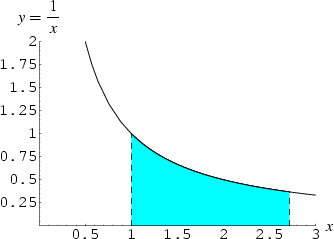Google’s IPO is valued at \$2,718,281,828. This is the value of the mathematical constant e (2.718281828…). Apparently they forgot to tack on 45 cents.

For the less mathematically inclined e is the base for the natural logarithm. It was chosen to honor Euler, one of the greatest mathematicians ever. It has the unique property such that the area of the region under the hyperbola `y = 1/x` from `x = 1` to `x = e` is exactly 1.

Here’s a mnemonic to memorize the first 40 digits of e…

“We present a mnemonic to memorize a constant so exciting that Euler exclaimed: ‘!’ when first it was found, yes, loudly ‘!’. My students perhaps will compute e, use power or Taylor series, an easy summation formula, obvious, clear, elegant!”